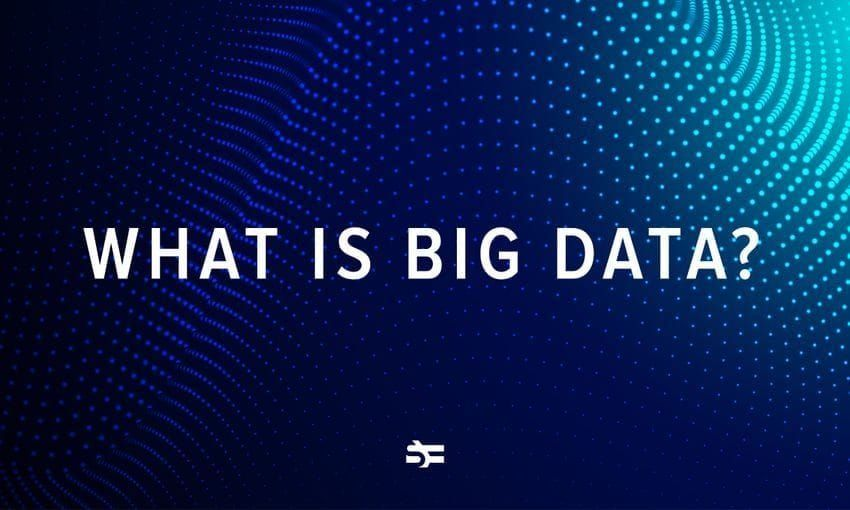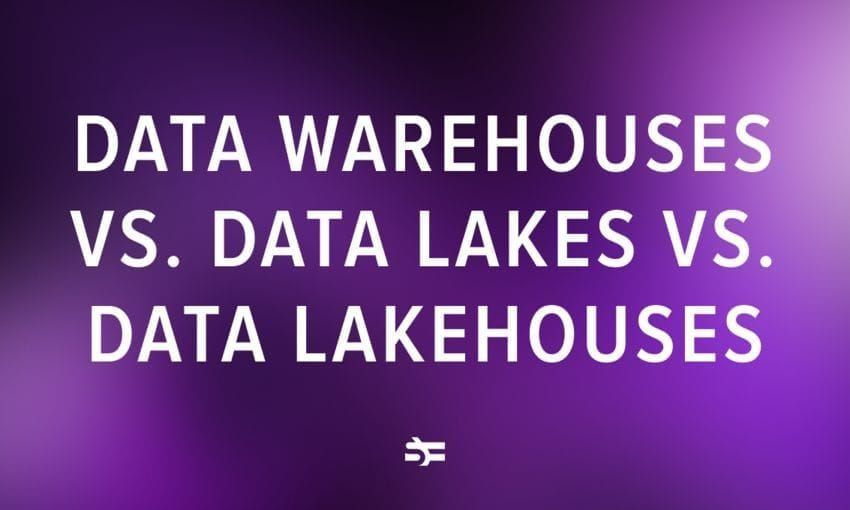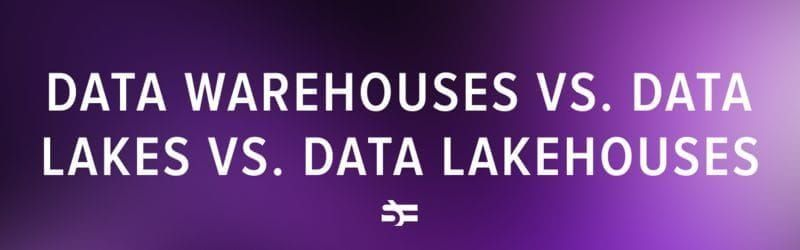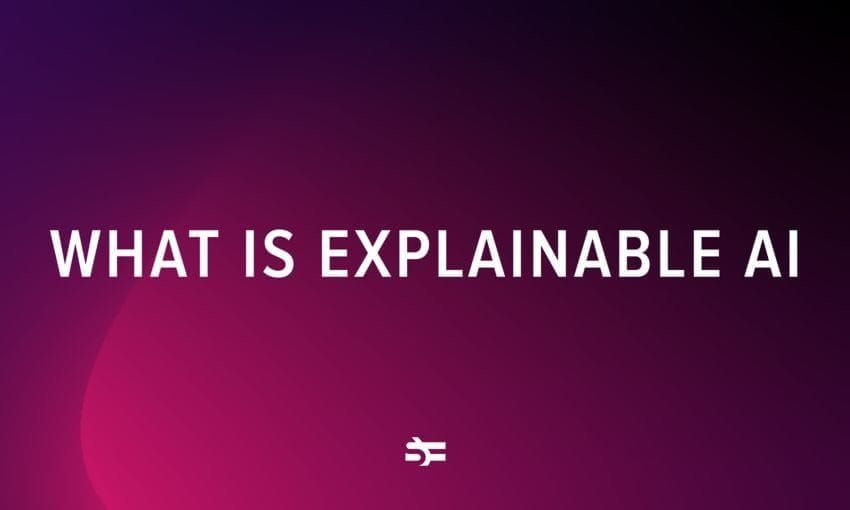# Introduction to Polynomial Regression Analysis

Polynomial regression is one of the machine learning algorithms used for making predictions. For example, it is widely applied to predict the spread rate of COVID-19 and other infectious diseases.

## What is regression analysis?Regression analysis is a helpful statistical tool for studying the correlation between two sets of events, or, statistically speaking, variables ― between a dependent variable and one or more independent variables. For example, your weight loss (dependent variable) depends on the number of hours you spend in the gym (independent variable).

There are several variations of statistical regression models.

### Simple linear regression

This type of regression model allows you to estimate the linear correlation between two variables, similar to the example above. Usually, the more time you spend on physical activity, the bigger your weight loss is; therefore, there is a linear correlation here. You can read more about simple linear regression in our blog post.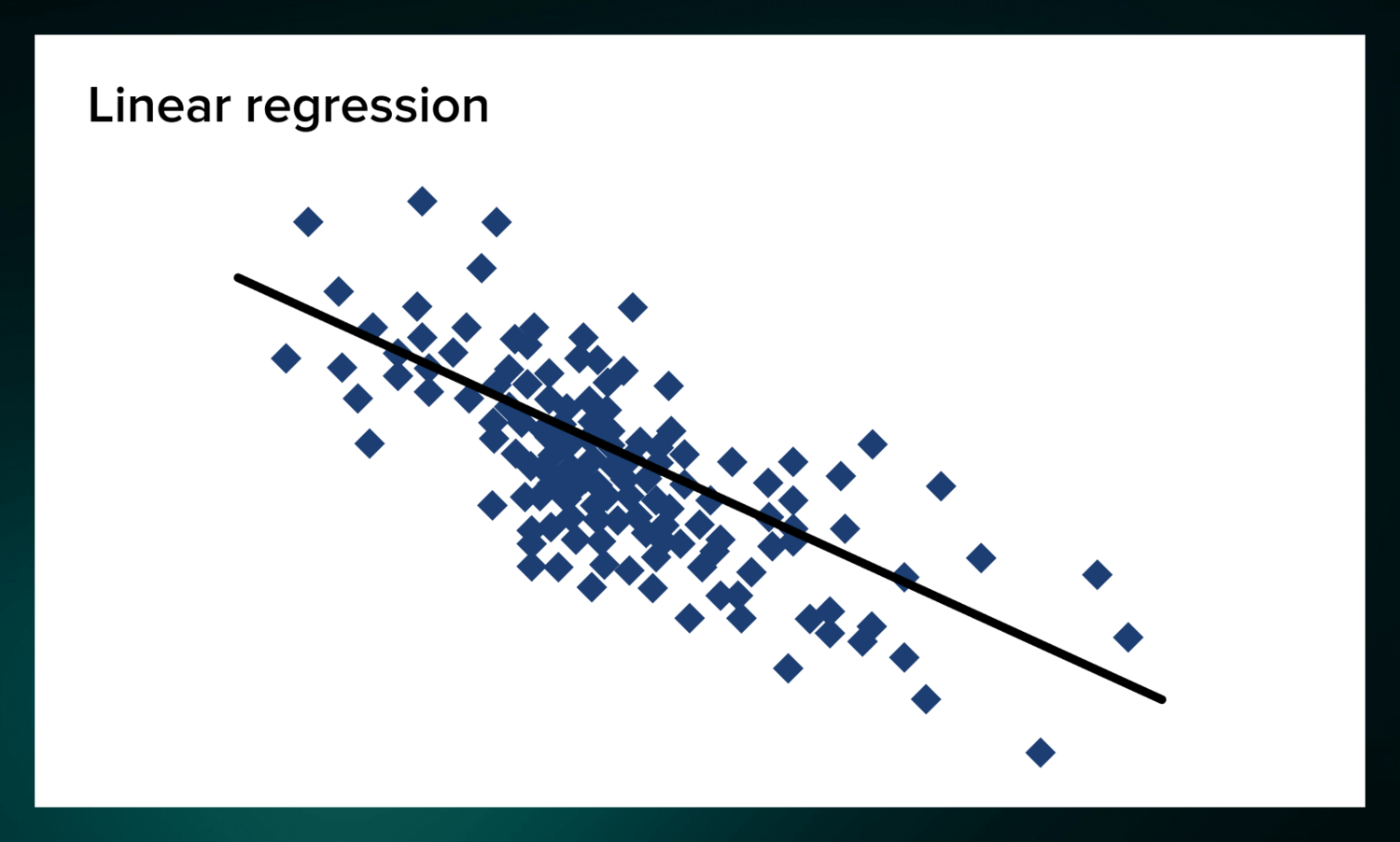### Multiple linear regression

Multilinear regression is related to simple linear regression. But instead of showing the correlation between just one dependent and one independent variable, you can consider several independent variables. For example, you can consider hours at the gym, daily sugar intake, and calories consumed to predict weight loss.

### Polynomial regression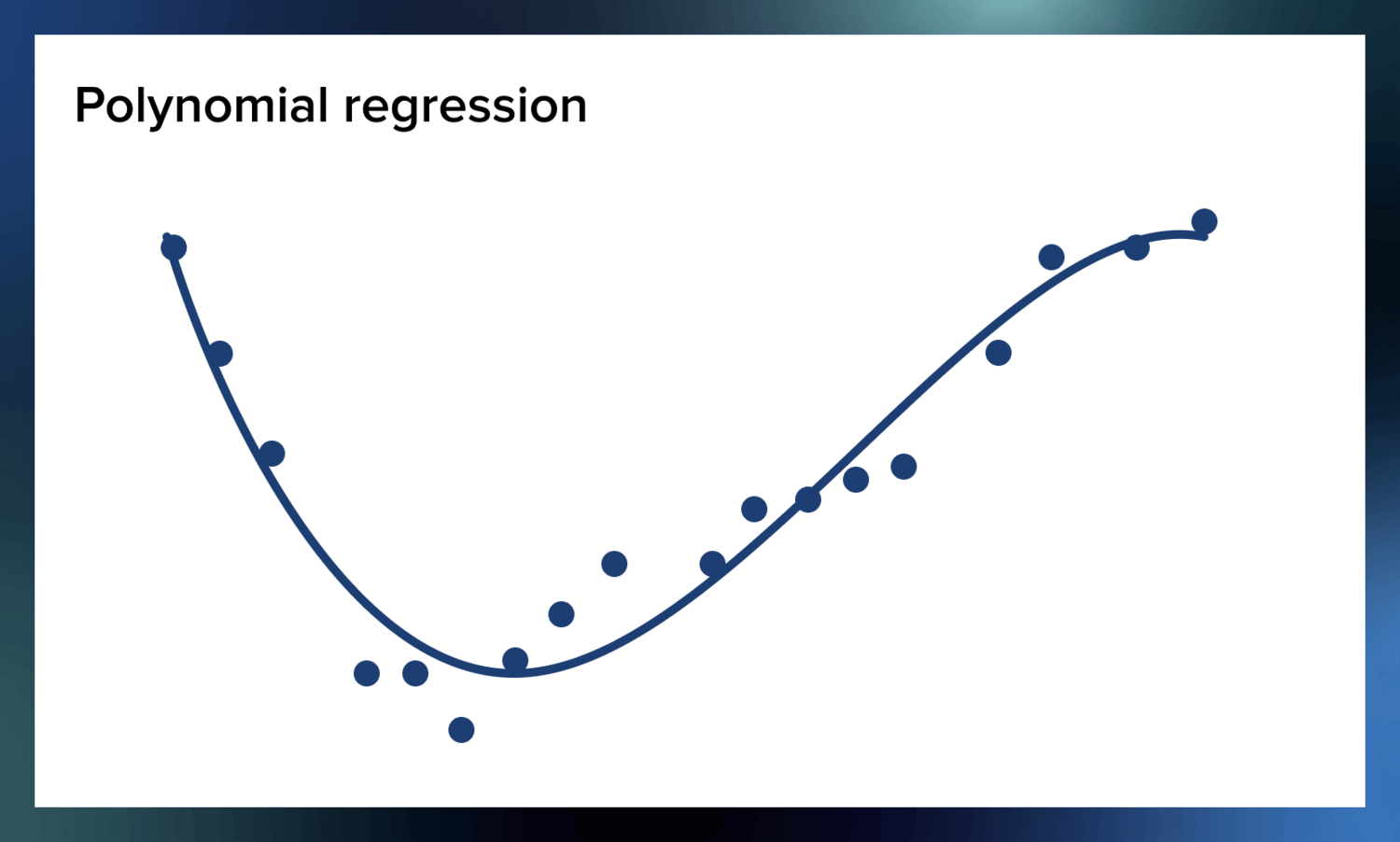Polynomial regression is needed when there is no linear correlation fitting all the variables. So instead of looking like a line, it looks like a nonlinear function. Let’s delve deeper into this type of regression.

## What is polynomial regression in machine learning?

Like many other things in machine learning, polynomial regression as a notion comes from statistics. Statisticians use it to conduct analysis when there is a non-linear relationship between the value of $x$ and the corresponding conditional mean of $y$.

Imagine you want to predict how many likes your new social media post will have at any given point after the publication. There is no linear correlation between the number of likes and the time that passes. Your new post will probably get many likes in the first 24 hours after publication, and then its popularity will decrease.

## Math behind polynomial regression

Here’s the general equation for a polynomial regression model.

$y= b_0+b_1x_1+ b_2{x_1}^2+ b_2{x_1}^3+ \ldots b_n{x_1}^n$

In the equation, $y$ is the dependent variable, $x$ is the independent variable, and $b_0$$b_n$ are the parameters you can optimize.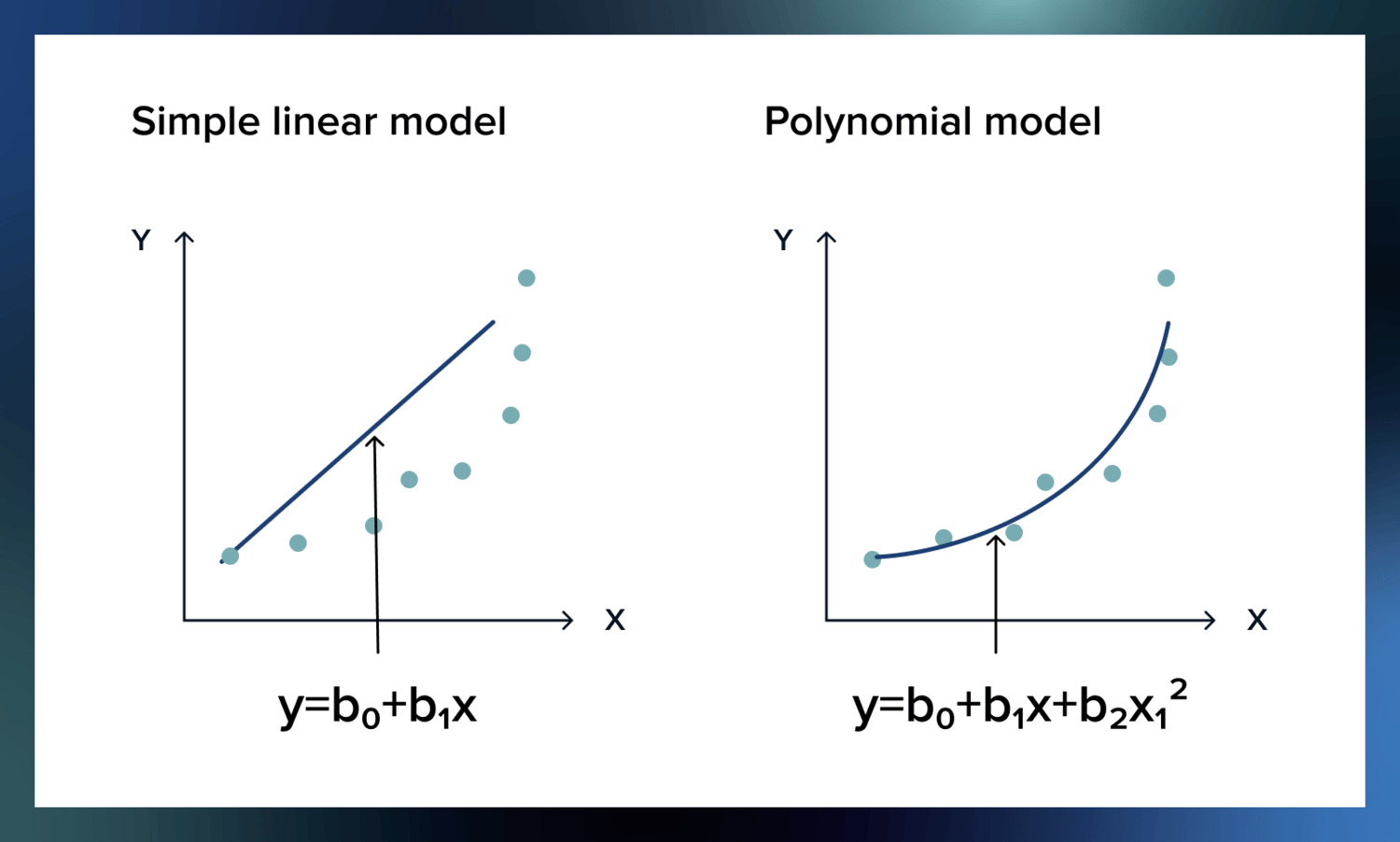Since the regression is linear in the parameters, you can fit the curve to your data by using the same methods you use for linear regressions – least squares and stuff.

Actually, as the sharp-eyed and the sharp-mathematically-minded might have noticed, this is just a special case of multiple linear regression.

Let’s repeat the example of weight loss.

In the case of multiple linear regression, you are interested in how multiple different values impact weight loss – like hours spent at the gym, sugar intake, and so on. In the case of polynomial regression, you are interested in how multiple different powers of one variable impact it. ($x$, $x^2$, $x^3$, and so on, where $x$ is the sugar intake, for example.)

Even though the curve will be bent in the second case, the statistical estimation problem is the same in both cases.

## Why do we need polynomial regression in ML?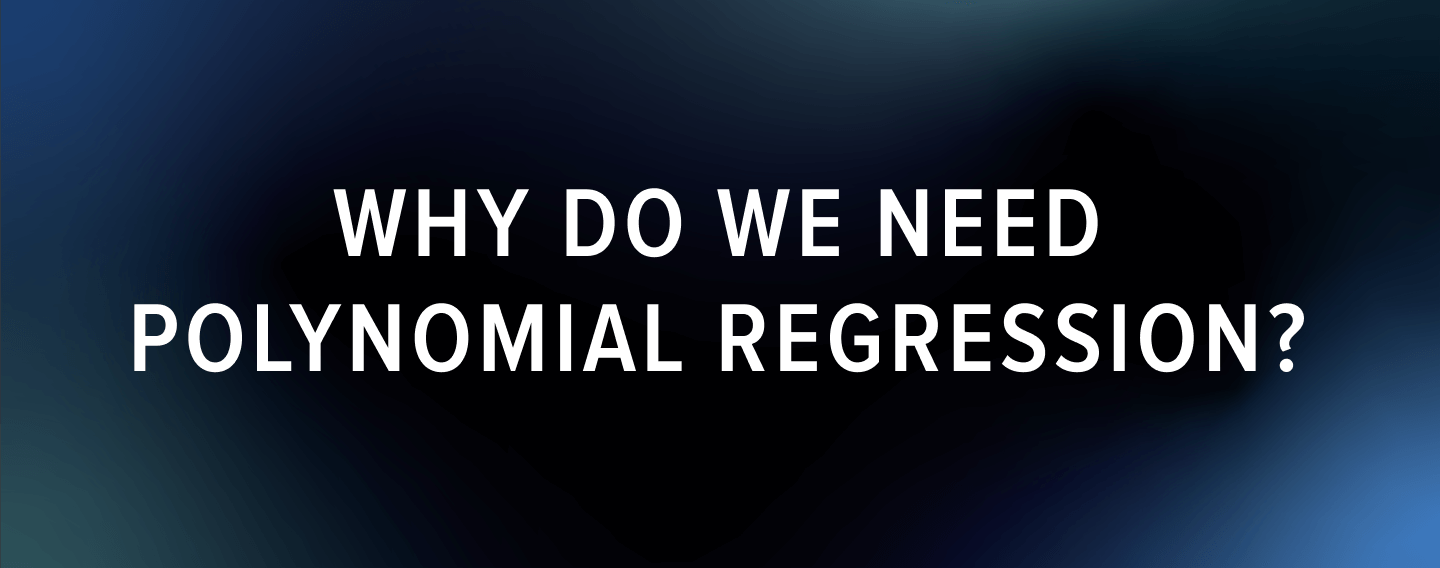Polynomial regression is useful in many cases. Since a relationship between the independent and dependent variables isn’t required to be linear, you get more freedom in the choice of datasets and situations you can be working with. So this method can be applied when simple linear regression underfits the data.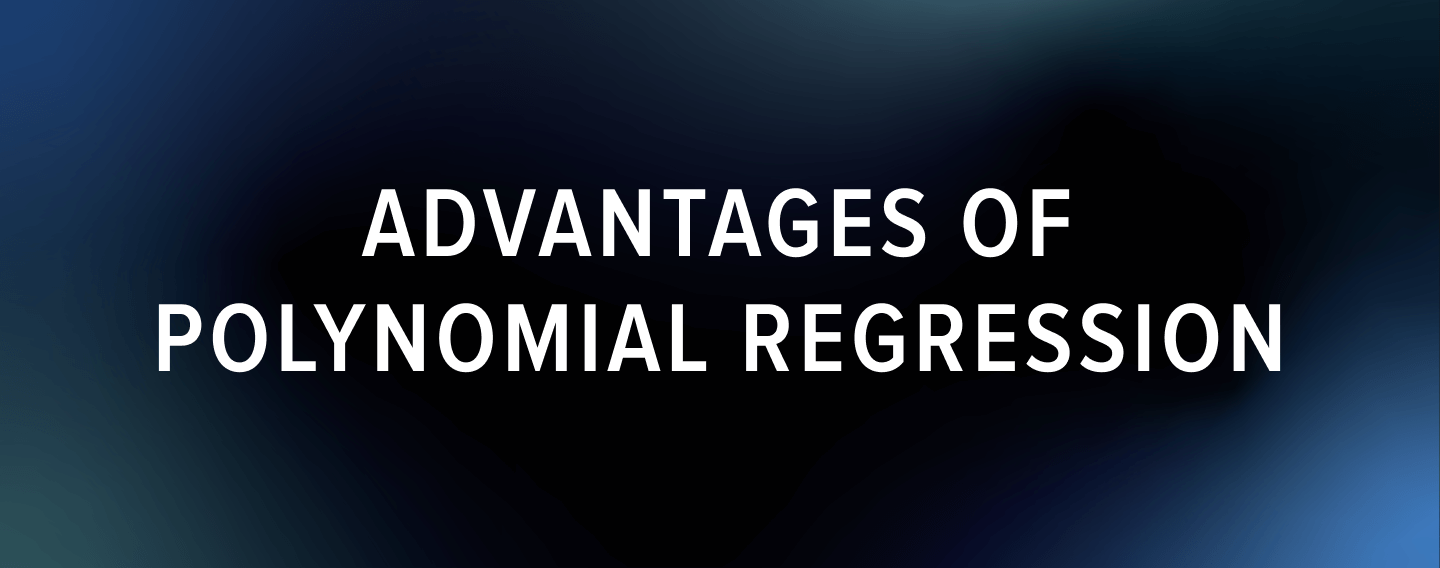Here are the pros of using polynomial regression for your next machine learning model:

• You can model non-linear relationships between variables.
• There is a large range of different functions that you can use for fitting.
• Good for exploration purposes: you can test for the presence of curvature and its inflections.

All in all, it is a flexible tool that can be used to fit a large variety of data point distributions.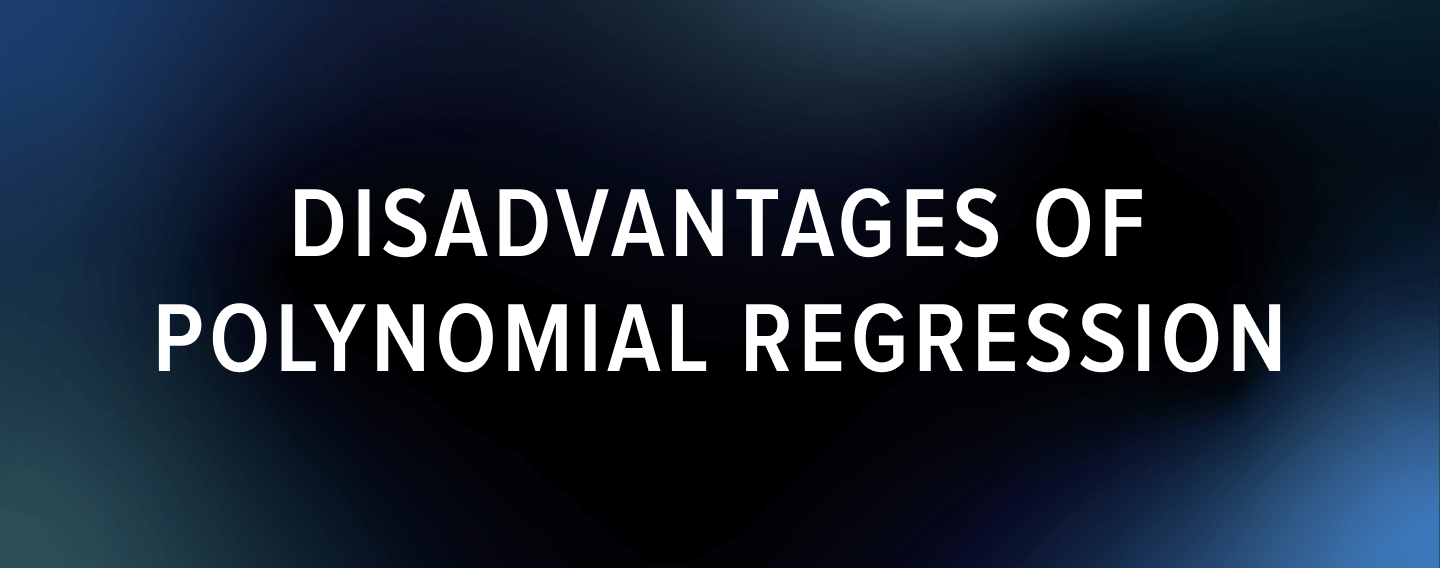However, just like linear regression, polynomial regression is not a universal tool.

• Even a single outlier in the data plot can seriously mess up the results.
• PR models are prone to overfitting. If enough parameters are used, you can fit anything. As John von Neumann reportedly said: “with four parameters I can fit an elephant, with five I can make him wiggle his trunk.”
• As a consequence of the previous, PR models might not generalize well outside of the data used.

## Where is polynomial regression used in machine learning?Now let us have a look at some practical examples where polynomial regression is used.

### Death rate prediction

When accidents happen, such as epidemics, fires, or tsunamis, it is important for catastrophe management teams to predict the number of injured or passed away people so that they can manage resources. It may take days, if not months, to mitigate the consequences of such events, and the team must be prepared. Polynomial regression allows us to build flexible machine learning models that report the potential death rate by analyzing many dependent factors. For example, in COVID-19 pandemics, these factors can be whether the patient has any chronic diseases, how often they are exposed to being in large groups of people, whether they have access to protective equipment, etc.

### Tissue growth rate prediction

Tissue growth rate prediction is used in different cases. Firstly, polynomial regression is often used to monitor oncology patients and the spread of their tumors. This type of regression helps to develop a model that considers the non-linear character of this spreading.

However, tissue growth rate prediction is also used in monitoring ontogenetic growth; in other words, it enables doctors to monitor the development of the organism in the womb from a very early stage.

### Speed regulation software

Today more and more speed regulation software systems powered by ML are aimed not at punishing violators of road conduct but at preventing unsafe behavior. Predictive modeling with the help of polynomial regression allows you to search for patterns in driver behavior and inform them about the necessity to adhere to the rules even before they overtake the speed limit. Preventative measures have been reported to be more effective and decrease the number of accidents on the roads.

## Final words

Polynomial regression is a simple yet powerful tool for predictive analytics. It allows you to consider non-linear relations between variables and reach conclusions that can be estimated with high accuracy. This type of regression can help you predict disease spread rate, calculate fair compensation, or implement a preventative road safety regulation software.

If you are looking for an expert team for machine learning project development, Serokell is there for you. Contact us to learn about our custom software solutions.

Stay tuned to our blog for more great materials about machine learning. In the meanwhile, if you feel adventurous, explore these materials about ML and regression:More from Serokell